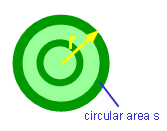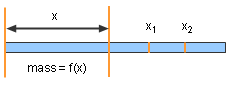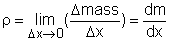Ch 2. Derivatives I Multimedia Engineering Math DerivativeFormulas TrigonometricFunctions Differentiability ChainRule ImplicitDifferentiation
 Chapter 1. Limits 2. Derivatives I 3. Derivatives II 4. Mean Value 5. Curve Sketching 6. Integrals 7. Inverse Functions 8. Integration Tech. 9. Integrate App. 10. Parametric Eqs. 11. Polar Coord. 12. Series Appendix Basic Math Units Search eBooks Dynamics Fluids Math Mechanics Statics Thermodynamics Author(s): Hengzhong Wen Chean Chin Ngo Meirong Huang Kurt Gramoll ©Kurt GramollMATHEMATICS - CASE STUDY SOLUTIONCircular Ripple A football is kicked into a pool. It creates a circular ripple that traveles outward with a speed of 2 ft/sec. How fast does the circular area enlarge after 3 seconds? If the radius of the circular ripple is r and the area of the circular ripple is s. The area of the circular ripple is      s = πr2 The enlarging circular area with respect to time t is s't or ds/dt      ds/dt = d(πr2)/dt              = d(π(r2))/dt              = 2πr(dr/dt) When t = 3 seconds,      r = (2)(3) = 6 So      ds/dt = 2πr(dr/dt)          = 2π(6)(2)          = 24π ft2/sec DiscussionNon-homogeneous Linear Density Calculation In order to evaluate the rate of the enlarging circular area, the concept of derivative has been employed. Derivative is widely used in science and engineering research. For example, how to calculate the linear density of a non-homogeneous rod. The mass from the left end to the point x is      mass = f(x) The mass between x2 and x1 is      Δmass = f(x2) - f(x1) So the average density of the rod between x2 and x1 is      average density = Δmass/Δx = ( f(x2) - f(x1))/(x2-x1) Letting x2 approaches x1, the linear density ρ of the rod at point x1 is the limit of the average density when Δx approaches 0.In other words, the linear density of the rod is the derivative of the mass with respect to length. Since the concept of derivative can apply to many problems in science and engineering, it is important for students to understand its physical meaning.